Chapter 22

The electric charge density wave and magnetic charge density wave are kinds of sound waves. The wave speed has an inverse relationship with the characteristic of electromagnetic impedance.

The characteristic electromagnetic impedance of a vacuum is a constant: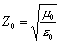(22.1)

What is the characteristic impedance of electron? From the equation (20.7), in Quantum Hall effect, the characteristic impedance of two dimensional electron gases is the ratio of the magnetic charge and electric charge.

Let us make an assumption that the electron characteristic impedance is the ratio of the magnetic charge and electric charge along with the spin direction. Let us now calculate the ratio of magnetic charge and electric charge inside the electron as follows:

Based on the equation (2.7), we have the electron’s electric charge in the sphere of radius ‘r’: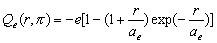(22.2)

The electric charge has the same distribution in both the north pole and south pole.
In the north pole area of sphere of radius ‘r,’ the electric charge is: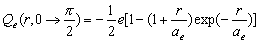(22.3)

In the south pole area of the electron sphere of radius ‘r’, the electric charge is: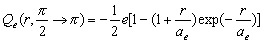(22.4)

Based on the equation (3.7), the magnetic charge distribution in a spher
e of radius ‘r’ is zero: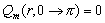(22.5)

In the north pole area of sphere of radius ‘r,’ the magnetic charge is: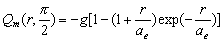(22.6)

The south pole area of sphere of radius ‘r,’ the magnetic charge is: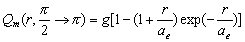(22.7)

Thus, within the north pole area of a sphere of any radius r, the ratio of magnetic charge and electric charge is: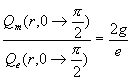(22.8)

The ratio is the constant, it doesn’t depend on the sphere radius ‘r’.

In the south pole area of sphere of radius r, the ratio of magnetic charge and electric charge is: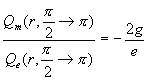(22.9)

The ratio is the constant; it doesn’t depend on the sphere radius ‘r’.

So we can see that inside the electron, for a sphere of any radius ‘r’, both the north pole and south pole have the same ratio of magnetic charge and electric charge, but with opposite signs.

Per our assumption that the electromagnetic characteristic impedance inside of an electron is the ratio of magnetic charge and electric charge along with the spin, which is: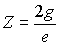(22.10)

As we know, ge=h, thus: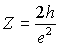(22.11)

As we know the fine structure constant is: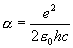(22.12)

Thus: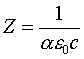(22.13)

Thus: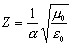(22.14)

Thus: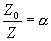(22.15)

The ratio of vacuum impedance and electron impedance is the alpha fine structure constant.

As we know, the electromagnetic wave velocity varies inversely with the wave impedance, and we also know that the wave velocity in the vacuum is the speed of light, and then we have following relationship: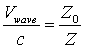(22.16)

Thus:(22.17)

Thus: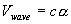(22.18)

As we know the velocity factor is the ratio of the speed of waves to the speed of light in a vacuum, we find out that the velocity factor of an electron is the alpha fine structure constant.

As we know, the ratio of magnetic charge unit and electric charge unit is as follows: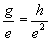(22.19)

As we can see, the value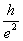is also the quantum hall resistance unit, which is also the ratio of the magnetic charge unit and electric charge unit.